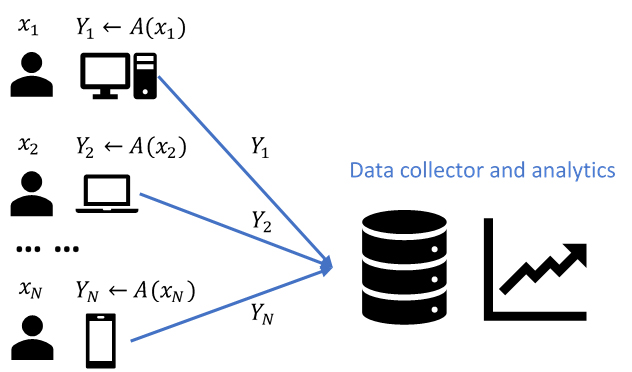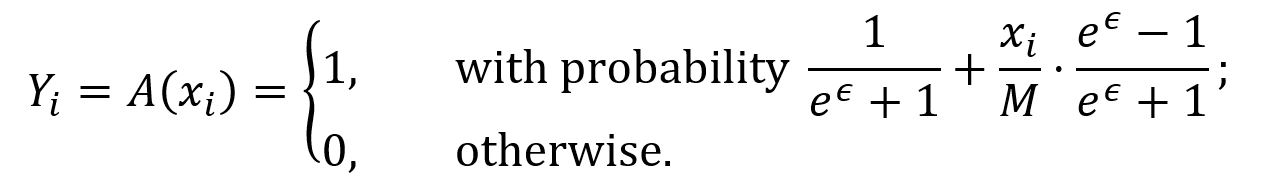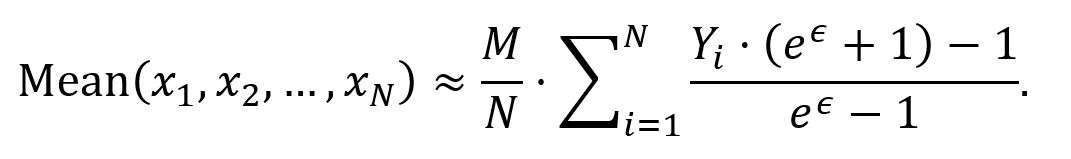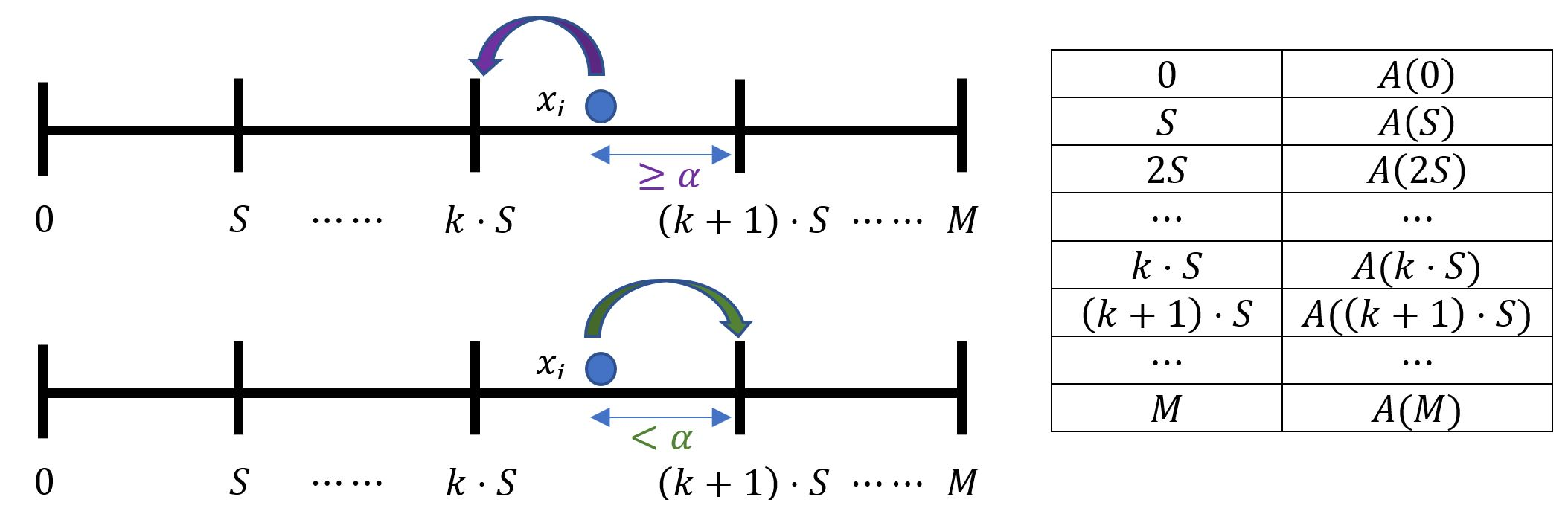# Microsoft Research Blog

## Collecting telemetry data privately

Published

By , Senior Researcher , Sr Principal Researcher

Research AreaThe collection and analysis of telemetry data from users and their devices leads to improved user experiences and informed business decisions. However, users have concerns about their data privacy, including what personal information software and internet companies are gathering and whether their data is protected from potential leaks and hacks.

Differential privacy (Dwork, et al. 2006) has emerged as the de facto standard for privacy guarantees. A special case (and the strongest variant) of differential privacy, called the local model, can be employed to address these concerns when collecting telemetry data. In the local model of differential privacy (LDP), each user holds a private telemetry value representing a characteristic such as time spent using a piece of software.

In the LDP model, instead of collecting xi directly, the data collector first runs a randomized algorithm A to encode xi on the user’s device, and then collects the output Yi = A(xi). LDP collection algorithm A needs to guarantee a type of plausible deniability: no matter what output A(x) is collected from a user, it is approximately equally as likely to have come from any specific value x as any other x’. Somewhat surprisingly, while observing values Yi = A(xi) coming from a large population of users, a data collector can tell almost nothing about any specific user, but can see general trends in the population, such as the mean, histogram, or other aggregates of users’ values.Figure 1. Data collection and analytics under the LDP model

One example of such LDP collection algorithms in our NIPS 2017 paper is the following 1-bit algorithm:Here a user with value xsends to the data collector Yi = 1 with probability that increases with xi /(assuming xi takes value from 0 to M) and Yi = 0 otherwise. The mean of {x1, x2, …, xN}  can be estimated asThe data collection algorithm A above satisfies ∈-local differential privacy (-LDP) because, for any two values x, x’ and [0, M] and b {0, 1}, we have Pr[A(x) = b] ≤ e ⋅ Pr[A(x’) = b].

The -LDP algorithm provides strong privacy guarantees for one-round data collection when  is small, e.g.,  = 1. Note that the smaller  is, the stronger the privacy guarantee provided, and the larger error in the resulting m  estimation. However, in practice, telemetry is typically collected continuously. What if, from each user, we want to collect the telemetry value for T continuous time stamps? We could apply the above algorithm A at each time stamp independently; however, based on the sequential composition of differential privacy, we end up with providing (T ⋅ )-LDP to each user. When T = 100, (100 ⋅ ∈) LDP is usually too weak to be a reasonable guarantee of privacy.

While memoization and instantaneous noise are useful techniques to protect consistent users in the face of repeated data collection (initially adopted by Erlingsson, et al. in RAPPOR for collecting strings under LDP), their efficacy depends on the fact that individual users take a constant number of distinct private values. Note that in our problem private values are real numbers. Thus, it is unreasonable to expect users to take consistent values across multiple rounds of data collection. In reality, users tend to be only approximately consistent: for example, software usage may vary by 30 minutes per day on most days. This leads to the following technical question:

How do we discretize continuous values so that we protect approximately consistent users while keeping the size of the memoization table small and losing no accuracy due to this discretization step?

Clearly, any deterministic discretization rule will incur some accuracy loss. Moreover, even if one is willing to tolerate errors due to rounding, such a rule would lead to a large memoization table, which may be difficult to implement in practice. We address this by making the rounding rule randomized.Figure 2. Rounding and memoization for repeated data collection

Our memoization scheme relies on α-point rounding—a technique that is used quite extensively in approximation algorithms for rounding linear programs. In this scheme, at the setup phase, every user draws value α from a uniform distribution over [0, S]; here S is the bucket size of discretization. We memoize output of our 1-bit algorithm A for values k ⋅ S, where k = 0,1,2, …, M/S k ⋅ S is a boundary between two consecutive buckets. At data collection, if the user takes a value xi such that k ⋅ S < xi  α  ≤ (k + 1) ⋅ S, then the user responds based the value k ⋅ S by looking up the memoization table.

Note that in our scheme, we also memoize the value of α, hence if a user is approximately consistent (the user’s values lie in a range with width S), then the output of our algorithm never changes or varies between two different values corresponding to neighboring buckets. Our rounding scheme also satisfies another desirable property: even with a discretization step, no matter how large the discretization size is, it introduces no further accuracy loss.

The ideas outlined here allow us to use memoization and instantaneous noise when collecting counter data. Our NIPS paper contains many more details, including discussion of privacy guarantees for inconsistent users, simultaneous collection of multiple counters, and more.

Related: